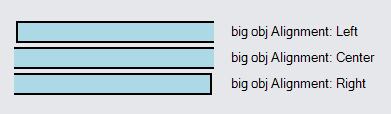# Sizing GraphObjects

The size of a GraphObject is determined by the values of the GraphObject.DesiredSize, GraphObject.MinSize, GraphObject.MaxSize and GraphObject.Stretch properties. The actual size of an object after its containing panel measures and arranges it is given by several read-only properties: GraphObject.NaturalBounds, GraphObject.MeasuredBounds, and GraphObject.ActualBounds.

The GraphObject.Width property is exactly the same as the Size.Width component of the GraphObject.DesiredSize. Similarly, the GraphObject.Height property corresponds to the DesiredSize's height. The default value of GraphObject.DesiredSize is `(NaN, NaN)` -- meaning that the size must be computed. One can set the width to a real number and leave the height to be `NaN`, or vice-versa.

Users can also change the size of an object within a Part via the ResizingTool: Introduction to the ResizingTool.

### DesiredSize, MinSize, and MaxSize

When the GraphObject.DesiredSize property is set to real numbers it gets that as its natural size. When the DesiredSize property is not set but there is a GraphObject.Stretch value, it will get the size of the available space. When DesiredSize is not set and there is no stretch, an object prefers being its natural size, based on the type of object that it is and the other properties that it has.

But the effective width and effective height, whether given by DesiredSize or computed, are each constrained by the GraphObject.MaxSize and by the GraphObject.MinSize. The minimum size takes precedence over the maximum size in case of conflict.

The size for a GraphObject in a Table Panel may also be constrained by the width of the column and the height of the row that the object is in.

``````
new Part("Table") { DefaultAlignment = Spot.Left }
new ColumnDefinition { Column = 0, Width = 200 },
new ColumnDefinition { Column = 1, Width = 15 }
)
new Shape {
Row = 0, Column = 0, Fill = "green",
Width = 100, Height = 20
},
new TextBlock("DesiredSize: 100x20, no MinSize, no MaxSize") {
Row = 0, Column = 2
},
new Shape {
Row = 1, Column = 0, Fill = "red",
Width = 100, Height = 20,
MinSize = new Size(150, 10)
},
new TextBlock("Desired: 100x20, Min: 150x10") {
Row = 1, Column = 2
},
new Shape {
Row = 2, Column = 0, Fill = "yellow",
Width = 100, Height = 20,
MaxSize = new Size(50, 300)
},
new TextBlock("Desired: 100x20, Max: 50x300") {
Row = 2, Column = 2
},
new Shape {
Row = 3, Column = 0, Fill = "red",
Width = 100, Height = 20,
MinSize = new Size(150, 10), MaxSize = new Size(50, 300)
},
new TextBlock("Desired: 100x20, Min: 150x10, Max: 50x300") {
Row = 3, Column = 2
}
)
);
``````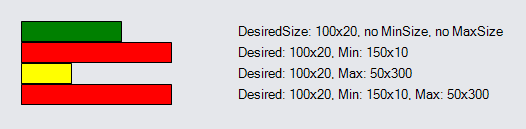### Measured and Actual Sizes

Every GraphObject also has a GraphObject.MeasuredBounds, which describes how big the object seems to be, and a GraphObject.ActualBounds, which describes the position and size of an object. These read-only properties take into account any non-zero GraphObject.Angle or non-unitary GraphObject.Scale. These measurements are in the containing Panel's coordinate system.

``````
string getSizeString(object o) {
var s = (Rect)o;
return s.Width.ToString("N2") + "x" + s.Height.ToString("N2");
}
var table =
new Part("Table")
new Shape("Diamond") {
Name = "A", Row = 0, Column = 1,
Fill = "green", Background = "lightgray",
Width = 40, Height = 40
},  // default angle is 0; default scale is 1
new Shape("Diamond") {
Name = "B", Row = 0, Column = 2,
Fill = "green", Background = "lightgray",
Width = 40, Height = 40,
Angle = 30
},
new Shape("Diamond") {
Name = "C", Row = 0, Column = 3,
Fill = "green", Background = "lightgray",
Width = 40, Height = 40,
Scale = 1.5
},
new Shape("Diamond") {
Name = "D", Row = 0, Column = 4,
Fill = "green", Background = "lightgray",
Width = 40, Height = 40,
Angle = 30, Scale = 1.5
},
new TextBlock { Row = 1, Column = 1, Margin = 4 }
.Bind(new Binding("Text", "NaturalBounds", getSizeString).OfElement("A")),
new TextBlock { Row = 1, Column = 2, Margin = 4 }
.Bind(new Binding("Text", "NaturalBounds", getSizeString).OfElement("B")),
new TextBlock { Row = 1, Column = 3, Margin = 4 }
.Bind(new Binding("Text", "NaturalBounds", getSizeString).OfElement("C")),
new TextBlock { Row = 1, Column = 4, Margin = 4 }
.Bind(new Binding("Text", "NaturalBounds", getSizeString).OfElement("D")),
new TextBlock { Row = 2, Column = 1, Margin = 4 }
.Bind(new Binding("Text", "MeasuredBounds", getSizeString).OfElement("A")),
new TextBlock { Row = 2, Column = 2, Margin = 4 }
.Bind(new Binding("Text", "MeasuredBounds", getSizeString).OfElement("B")),
new TextBlock { Row = 2, Column = 3, Margin = 4 }
.Bind(new Binding("Text", "MeasuredBounds", getSizeString).OfElement("C")),
new TextBlock { Row = 2, Column = 4, Margin = 4 }
.Bind(new Binding("Text", "MeasuredBounds", getSizeString).OfElement("D")),
new TextBlock { Row = 3, Column = 1, Margin = 4 }
.Bind(new Binding("Text", "ActualBounds", getSizeString).OfElement("A")),
new TextBlock { Row = 3, Column = 2, Margin = 4 }
.Bind(new Binding("Text", "ActualBounds", getSizeString).OfElement("B")),
new TextBlock { Row = 3, Column = 3, Margin = 4 }
.Bind(new Binding("Text", "ActualBounds", getSizeString).OfElement("C")),
new TextBlock { Row = 3, Column = 4, Margin = 4 }
.Bind(new Binding("Text", "ActualBounds", getSizeString).OfElement("D")),
new TextBlock("NaturalBounds:") { Row = 1, Column = 0, Alignment = Spot.Left },
new TextBlock("MeasuredBounds:") { Row = 2, Column = 0, Alignment = Spot.Left },
new TextBlock("ActualBounds:") { Row = 3, Column = 0, Alignment = Spot.Left }
);

// cause bindings to be evaluated after Shapes are measured
table.Data = new { };
});
``````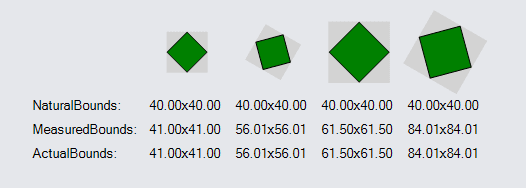Note that the size of the regular 40x40 shape is 41x41. The additional size is due to the thickness of the pen (Shape.StrokeWidth) used to outline the shape. Rotating or increasing the scale causes the 40x40 shape to actually take up significantly more space.

To summarize: the GraphObject.DesiredSize (a.k.a. GraphObject.Width and GraphObject.Height) and the GraphObject.NaturalBounds are in the object's local coordinate system. The GraphObject.MinSize, GraphObject.MaxSize, GraphObject.Margin, GraphObject.MeasuredBounds, and GraphObject.ActualBounds are all in the containing Panel's coordinate system, or in document coordinates if there is no such panel because it is a Part.

### Stretching of GraphObjects

When you specify a GraphObject.Stretch value other than Stretch.None, the object will stretch or contract to fill the available space. However, the GraphObject.MaxSize and GraphObject.MinSize properties still limit the size.

But setting the GraphObject.DesiredSize (or equivalently, the GraphObject.Width and/or GraphObject.Height) will cause any stretch value to be ignored.

In the following examples the left column is constrained to have a width of 200.

``````
new Part("Table") { DefaultAlignment = Spot.Left }
new ColumnDefinition { Column = 0, Width = 200 },
new ColumnDefinition { Column = 1, Width = 15 }
)
new Shape {
Row = 0, Column = 0, Fill = "green",
Stretch = Stretch.Fill
},
new TextBlock("Stretch.Fill, no MinSize, no MaxSize") {
Row = 0, Column = 2
},
new Shape {
Row = 1, Column = 0, Fill = "red",
Stretch = Stretch.Fill,
MinSize = new Size(150, 10)
},
new TextBlock("Stretch.Fill, Min: 150x10") {
Row = 1, Column = 2
},
new Shape {
Row = 2, Column = 0, Fill = "yellow",
Stretch = Stretch.Fill,
MaxSize = new Size(50, 300)
},
new TextBlock("Stretch.Fill, Max: 50x300") {
Row = 2, Column = 2
},
new Shape {
Row = 3, Column = 0, Fill = "red",
Stretch = Stretch.Fill,
MinSize = new Size(150, 10), MaxSize = new Size(50, 300)
},
new TextBlock("Stretch.Fill, Min: 150x10, Max: 50x300") {
Row = 3, Column = 2
},
new Shape {
Row = 4, Column = 0, Fill = "red",
Width = 100, Stretch = Stretch.Fill
},
new TextBlock("desired width & Stretch: ignore Stretch") {
Row = 4, Column = 2
}
)
);
``````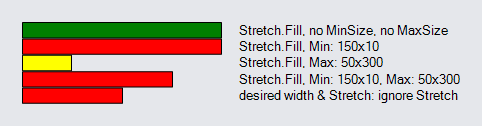To summarize, if GraphObject.DesiredSize is set, any GraphObject.Stretch is ignored. If GraphObject.MaxSize conflicts with that value, it takes precedence. And if GraphObject.MinSize conflicts with those values, it takes precedence. The width values are constrained independently of the height values.

## Stretch and Alignment

The size of a GraphObject in a Panel is determined by many factors. The GraphObject.Stretch property specifies whether the width and/or height should take up all of the space given to it by the Panel. When the width and/or height is not stretched to fill the given space, the GraphObject.Alignment property controls where the object is placed if it is smaller than available space. One may also stretch the width while aligning vertically, just as one may also stretch vertically while aligning along the X axis.

The alignment value for a GraphObject, if not given by the value of GraphObject.Alignment, may be inherited. If the object is in a Table Panel, the value may inherit from the Row/ColumnDefinitions of the row and of the column that the object is in. Finally the value may be inherited from the Panel.DefaultAlignment property.

If you specify a fill stretch (horizontal or vertical or both) and an alignment, the alignment will be ignored. Basically if an object is exactly the size that is available to it, there is only one position for it, so all alignments are the same.

### Alignment of Shapes

``````
new Part("Table") { DefaultAlignment = Spot.Left }
new ColumnDefinition { Column = 0, Width = 200 },
new ColumnDefinition { Column = 1, Width = 15 }
)
new Shape {
Row = 0, Column = 0, Fill = "lightblue",
Width = 100, Height = 20, Alignment = Spot.Left
},
new TextBlock("Alignment: Left") { Row = 0, Column = 2 },
new Shape {
Row = 1, Column = 0, Fill = "lightblue",
Width = 100, Height = 20, Alignment = Spot.Center
},
new TextBlock("Alignment: Center") { Row = 1, Column = 2 },
new Shape {
Row = 2, Column = 0, Fill = "lightblue",
Width = 100, Height = 20, Alignment = Spot.Right
},
new TextBlock("Alignment: Right") { Row = 2, Column = 2 },
new Shape {
Row = 3, Column = 0, Fill = "yellow",
Height = 20, Stretch = Stretch.Horizontal
},
new TextBlock("Stretch: Horizontal") { Row = 3, Column = 2 },
new Shape {
Row = 4, Column = 0, Fill = "yellow",
Height = 20, Stretch = Stretch.Horizontal, Alignment = Spot.Right
},
new TextBlock("Stretch: Horizontal, ignore Alignment") { Row = 4, Column = 2 }
)
);
``````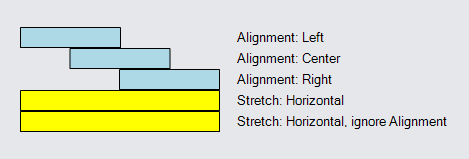When the element is larger than the available space, the GraphObject.Alignment property still controls where the element is positioned. However the element will be clipped to fit.

To make things clearer in the following example we have made the shape stroke thicker and added a margin to separate the shapes.

``````
new Part("Table") { DefaultAlignment = Spot.Left }
new ColumnDefinition { Column = 0, Width = 200 },
new ColumnDefinition { Column = 1, Width = 15 }
)
new Shape {
Row = 0, Column = 0, Fill = "lightblue", StrokeWidth = 2,
Width = 300, Height = 20, Margin = 2, Alignment = Spot.Left
},
new TextBlock("big obj Alignment: Left") { Row = 0, Column = 2 },
new Shape {
Row = 1, Column = 0, Fill = "lightblue", StrokeWidth = 2,
Width = 300, Height = 20, Margin = 2, Alignment = Spot.Center
},
new TextBlock("big obj Alignment: Center") { Row = 1, Column = 2 },
new Shape {
Row = 2, Column = 0, Fill = "lightblue", StrokeWidth = 2,
Width = 300, Height = 20, Margin = 2, Alignment = Spot.Right
},
new TextBlock("big obj Alignment: Right") { Row = 2, Column = 2 }
)
);
``````